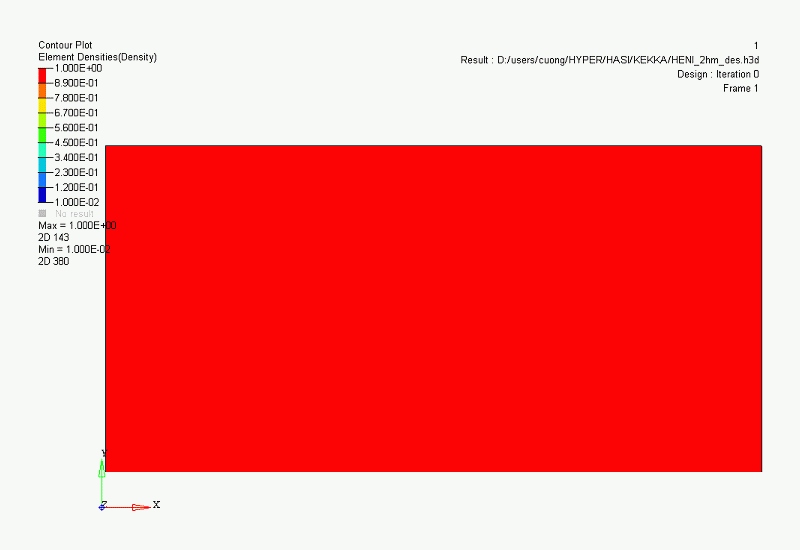# Topology Optimization

## Features

Topology optimization is a mathematical method to optimize the material layout under some constraints. Constraints such as design space, load conditions, constraint conditions, and “load point displacement is less than a few millimeters” are given, and the layout that maximizes the desired performance index is calculated.

In the design space model, find the optimum shape for the expected product usage environment and SPEC.
It is possible to reflect highly novel shapes and ideas that humans could not think of in product design.

## Calculation of the optimal shape that maximizes performance under constraint

Here is a simple example of topology optimization.
The green area on the left is the design space. In this space, an instruction is given for the shape to be “the lightest shape with a load point displacement of less than a” with the both ends fixed, and the loading is applied to three places.

The calculation process is shown on the right. The density of each part is changed in the design space to optimize the material arrangement.The red part is judged as necessary while the blue part is judged as unnecessary.In this case, a shape resembling an iron bridge was automatically calculated through 59 iterations.## Challenge for Ultra Light Weight Brake Pedal Brackets

This is an example of topology optimization from a steel model to a resin model to derive the optimal shape.

We support the design of high performance products in a short period of time and at low cost, through the use of CAE.